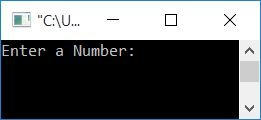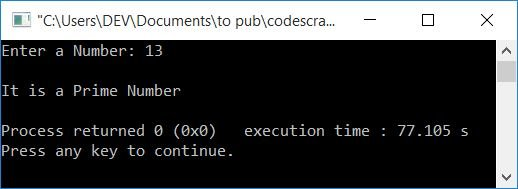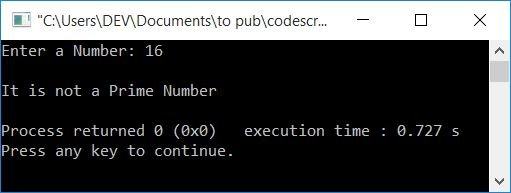# C++ program to check if a given number is a prime number or not

In this article, you will learn and get code on checking whether a given number, given by the user at run-time, is a prime number or not in C++. The program is created in the following ways:

### What is a prime number?

A prime number is a number that can only be divisible by 1 and the number itself. That is, if a number has no factors except 1 and the number itself, then it is called a prime number. For example, 13, 7, 17, 19, 23, etc.

## Examine the prime number in C++

To check whether the number is a prime number or not in C++ programming, you have to ask the user to enter a number first, and then check and print as shown in the program given below:

If a number, n, is divisible by any number from 2 to one less than the number (n-1), then it is not prime; otherwise, it is a prime number.

```#include<iostream>
using namespace std;
int main()
{
int num, i, chk=0;
cout<<"Enter a Number: ";
cin>>num;
for(i=2; i<num; i++)
{
if(num%i==0)
{
chk++;
break;
}
}
if(chk==0)
cout<<"\nIt is a Prime Number";
else
cout<<"\nIt is not a Prime Number";
cout<<endl;
return 0;
}```

This program was built and runs under the Code::Blocks IDE. Here is its sample run:Now supply any number as input, say 13, and press the ENTER key to check whether it is a prime or not, as shown here:The dry run of the above program (with user input 13) goes like this:

• Initially chk=0 and num=13 (the user entered 13).
• Inside the for loop, the initialization part executes first and only once. Therefore i=2
• Checks whether the value of i is less than the value of num or not.
• The condition evaluates to be true because 2 (the value of i) is less than 13 (the value of num), therefore program flow goes inside the loop.
• Inside the loop, there is an if statement that checks whether the value of num is divisible by the current value of i or not.
• Note: If the value of i ever divides num, just increment the value of count and exit the loop using the break keyword.
• Because 13 is not divisible by 2, the condition evaluates to be false, therefore program flow doesn't go inside the if's body.
• Now the program flow goes to the update part of the for loop and increments the value of i. Now i=3. Checks for the condition again to see if i<num or 3<13 evaluates to true or false.
• Condition again evaluates to be true, therefore program flow again goes inside the loop.
• In this manner, each time you enter the loop, check whether the num's value is divisible by the i's value.
• Continue the process until the condition of the for loop evaluates to be false.
• After exiting from the loop, check for the value of chk and print the message.
• That is, if chk holds its initial value (0), then the given number is a prime number, otherwise it is not a prime number.

## In C++, use a function to check the prime number

Now let's create the same-purpose program using a user-defined function called checkPrimeNumber(). It takes the number entered by the user as an argument and returns a value. Initialize its return value to a variable, say chk, so check its value and print the message accordingly.

```#include<iostream>
using namespace std;
int main()
{
int num, chk;
cout<<"Enter a Number: ";
cin>>num;
if(chk==0)
cout<<"\nIt is a Prime Number";
else
cout<<"\nIt is not a Prime Number";
cout<<endl;
return 0;
}
{
int i, chk=0;
for(i=2; i<num; i++)
{
if(num%i==0)
{
chk++;
return chk;
}
}
return chk;
}```

It will produce the same output as the previous program. Here is its sample run with user input as 16:You can also prefer the program given below for the same purpose as the previous one. In this program, rather than returning a value to the main() function, this program checks and prints directly from inside the function:

```#include<iostream>
using namespace std;
int main()
{
int num;
cout<<"Enter a Number: ";
cin>>num;
cout<<endl;
return 0;
}
{
int i, chk=0;
for(i=2; i<num; i++)
{
if(num%i==0)
{
chk++;
break;
}
}
if(chk==0)
cout<<"\nIt is a Prime Number";
else
cout<<"\nIt is not a Prime Number";
}```

## In C++, check the prime number using the class

This is the last program that uses classes and objects to check for the prime number. The question is: write a program in C++ to check whether an input number is a prime number or not using classes. Here is its answer:

```#include<iostream>
using namespace std;
class CodesCracker
{
private:
int num, i, chk;
public:
int getData();
};
int CodesCracker::getData()
{
cout<<"Enter a Number: ";
cin>>num;
return num;
}
{
int i, chk=0;
for(i=2; i<num; i++)
{
if(num%i==0)
{
chk++;
return chk;
}
}
return chk;
}
int main()
{
CodesCracker c;
int num, chk=0;
num = c.getData();
if(chk==0)
cout<<"\nIt is a Prime Number";
else
cout<<"\nIt is not a Prime Number";
cout<<endl;
return 0;
}```

The brief explanation of the above code is:

• A CodesCracker (class) object c is created.
• Using this object, we have called the function getData() of the class CodesCracker.
• and its return value gets initialized to num inside the main() function.
• Because the getdata() function receives a value from the user and returns it, the number entered by the user is basically initialized to num.
• Now we've passed num to checkPrimeNumber() function. This function checks whether it is a prime or not. It returns 0 (if num is a prime number) or 1 (if num is a composite number), and its return value gets initialized to chk inside the main() function.
• Finally, we have checked the value of chk and printed the message.

#### The same program in different languages

C++ Quiz

« Previous Program Next Program »